# Grade - examples - page 35

1. TilesThe room has dimensions 12 m and 5.6 m. Determine the number of square tiles and their largest possible size to cover them room's floor.
2. Dog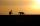Man goes with a dog on a long walk 17 km from the house. Man is walking at speed 4.9 km/h and a dog that constantly running between house and man at 12.6 km/h. How many kilometers will run the dog when they reach their destination?
3. Insurance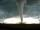The owner of the house is insured against natural disasters and pays 0.04% annually of the value of house 77 Eur. Calculate the value of the house. Calculate the probability of disaster, if you know that 48% of the insurance is to pay damages.
4. Isosceles IIIThe base of the isosceles triangle is 17 cm area 416 cm2. Calculate the perimeter of this triangle.
5. N-gon angles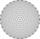What is the sum of interior angles 9-gon? What is the internal angle of a regular convex 9-polygon?
6. Rectangle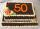Area of rectangle is 3002. Its length is 41 larger than the width. What are the dimensions of the rectangle?
7. Equilateral cylinderEquilateral cylinder (height = base diameter; h = 2r) has a volume of V = 199 cm3 . Calculate the surface area of the cylinder.
8. 2nd class combinationsFrom how many elements you can create 4560 combinations of the second class?
9. CuboidCuboid ABCDEFGH with 10 cm height has a base edge length 6 cm and 8 cm. Determine angle between body diagonal and the base plane (round to degrees).
10. Bits, bytes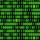Calculate how many different numbers can be encoded in 16-bit binary word?
11. Children poolThe bottom of the children's pool is a regular hexagon with a = 60 cm side. The distance of opposing sides is 104 cm, the height of the pool is 45 cm. A) How many liters of water can fit into the pool? B) The pool is made of a double layer of plastic film.
12. Garden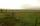The garden has two opposite parallel fences. Their distance is 33.1 m. Lengths in these two fences are 75.5 meters and 49.4 meters. Calculate the area of this garden.
13. Tetrahedral prismCalculate surface and volume tetrahedral prism, which has a rhomboid-shaped base, and its dimensions are: a = 12 cm, b = 7 cm, ha = 6 cm and prism height h = 10 cm.Convert magnitude of the angle α = 9°39'15" to radians:
15. Party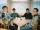At the party everyone clink with everyone. Together, they clink 406 times. How many people were at the party?
16. Horizon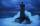The top of a lighthouse is 17 m above the sea. How far away is an object which is just “on the horizon”? [Assume the earth is a sphere of radius 6378.1 km.]Calculate the radius of the quadrant, which area is equal to area of circle with radius r = 15 cm.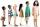How many different ways can sit 8 boys and 3 girls in line, if girls want to sit on the edge?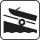A force of 300 kg (3000 N) is required to pull a boat up a ramp inclined at 14° with horizontal. How much does the boat weight?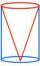The cylinder is inscribed cone. Determine the ratio of the volume of cone and cylinder. The ratio express as a decimal number and as percentage.